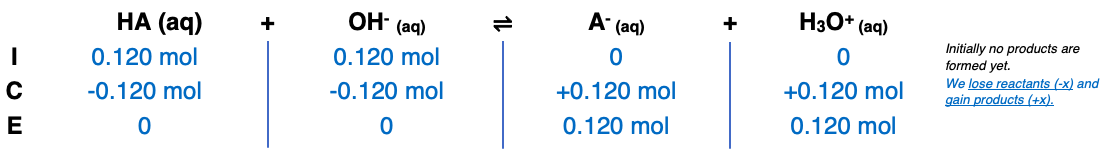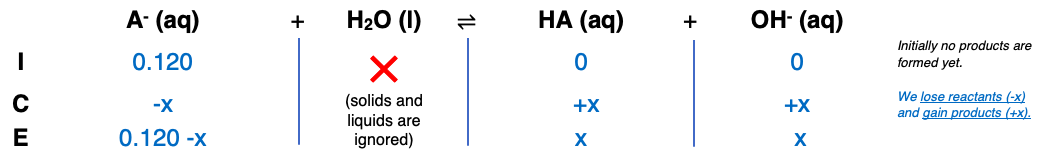# Problem: Calculate the pH at the equivalence point in titrating 0.120 M solutions of each of the following acids with a solution 0.090 M in NaOH.chlorous acid (HClO2)

###### FREE Expert Solution

Step 1Step 2Step 3###### Problem Details
Calculate the pH at the equivalence point in titrating 0.120 M solutions of each of the following acids with a solution 0.090 M in NaOH.

chlorous acid (HClO2)